# Analytic model of a language

In the most fully developed analytic models of languages, the set of initial data is usually an object which models a set of grammatical sentences of a natural language, viz. some formal language in a given alphabet (vocabulary). Ifis a language in the vocabularyand, then one says that the stringcan be substituted by the string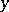with respect toand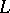; if each one the stringsandcan be substituted by the other with respect toand, then one says thatandcan be mutually substituted with respect toand. The concept of mutual substitutability has a simple linguistic meaning: Ifis understood to be the set of grammatical sentences of some natural language, the mutually substitutable sets are "syntactically equivalent" (i.e. fulfilling the same syntactic functions) word combinations. In particular, if a single-symbol string(in linguistic interpretation: a word) is mutually substitutable with a stringof length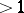,is a "potential constituent" , i.e. may be a constituent of the linguistically natural constituent structures of grammatical sentences of this language (cf. Syntactic structure); in such a case the stringis called a first rank configuration of the languagewith resultant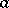. Thus, in the English language, the string "uniformly continuous" can be regarded as a first rank configuration with resultant "continuous" . However, first rank configurations do not exhaust all "potential constituents" ; for example, the word combination "continuous function" is not a first rank configuration, since only words such as "function" , "derivative" , etc., can be substituted by it, but it cannot itself be substituted by any of these words ( "fx is a uniformly continuous function" is a correct statement, but "fx is a uniform function" is not). Therefore one introduces the following definition: If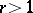is a natural number and if the concept of a rankconfiguration of the languagehas been defined for each, then a stringof lengthis called a rankconfiguration of the languagewith resultant, where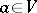, if the following condition holds: Ifcan be substituted bywith respect toand, if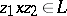and if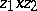does not contain occurrances of configurations of ranks lower thanoverlapping the consideredbut not entirely contained in it, then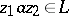. In English, the word combination "continuous function" can be regarded as a second rank configuration, the word "function" being the resultant (the word "derivative" , for example, is also a resultant). It may be shown that, in a certain sense, a language is fully determined by the set of its configurations.
The configurational model belongs to the so-called syntagmatic analytic models of a language, which are intended to describe the relations between the elements of segments of speech (in linguistics such relations are called syntagmatic). Another class of analytic models of a language are paradigmatic models, intended to describe paradigmatic relations, i.e. relations between the elements of a language in its system. In such models the usual procedure is to construct certain relations on the vocabulary, which are often (but not always) equivalence relations. In particular, paradigmatic analytic models of a language serve to construct formal analogues of traditional linguistic categories such as parts of speech, case, gender, phoneme, etc. A set of grammatical sentences also serves as the "starting material" in many paradigmatic analytic models of a language; the concept of substitutability is employed in several models of this type. The simplest equivalence relation obtained in this way is the mutual substitutability relation on the vocabulary (i.e. on the set of all one-element strings); the equivalence classes induced by this relation are called families. If one introduces an additional relation on the vocabulary, consisting of "to be different forms of the same word" (more precisely, of one lexeme; this is, e.g., the relation between "to limit" and "limiting" or between "number" and "numbers" ; it involves a certain idealization in that it is assumed that a word may be the form of one lexeme only; the respective equivalence classes are called neighbourhoods), then these two relations may be used to introduce certain other classifications, which may be regarded as approximations to the formal analogues of parts of speech and other traditional grammatical concepts, in particular the concepts of case and gender of nouns. These last two concepts are particularly intensively studied; a number of models based on the concept of grammaticality as well as other types of models have been suggested for their formalization. In one model, in particular, each case of nouns is treated as a set of "uniformly directed" forms of nouns, while each gender is treated as a set of "uniformly directing" nouns. The initial data in this model, which serve to determine the gender, are the vocabulary, the set of neighbourhoods, the set of nouns and the binary relation of "potential subordination" in the vocabulary:potentially subordinatesif, in some "linguistically natural" dependency structure (cf. Syntactic structure) of a grammatical sentence in this language, some occurrence of(immediately) depends on some occurrence of; additional initial data of the same nature are added to determine the gender. Models based on "potential subordination" or other concepts related to dependency structures apparently permit a better formalization of traditional grammatical categories than do models involving strings only, since in the former models syntactic and linear relations are separated (cf. Grammar, transformational).